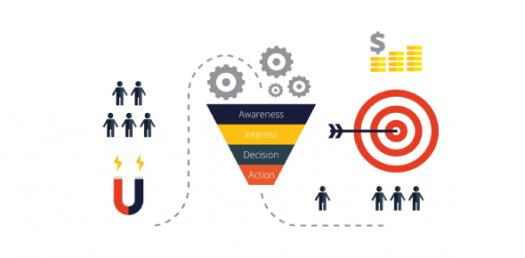Volume And Capacity Units Conversion Quiz

8 Questions | Total Attempts: 1668SettingsTake this quiz to make sure you know how to convert units of volume and capacity!

• 1.
How many  are there in 1.86  ?
• A.

18600000

• B.

1860000

• C.

186000

• D.

1860

• E.

186

• 2.
How many  are there in   ?
• A.

0.045

• B.

45

• C.

450

• D.

450000

• E.

450000000

• 3.
Convert 6.8 L to mL.
• A.

0.0068

• B.

6.8

• C.

680

• D.

6800

• E.

68000000

• 4.
How many  are there in   ?
• A.

0.332

• B.

0.0332

• C.

3.32

• D.

3320

• E.

332000

• 5.
Convert   to  .
• A.

47

• B.

0.000047

• C.

470

• D.

4700

• E.

47000

• 6.
How many  are there in  ?
• A.

0.288856

• B.

288856000

• C.

288.856

• D.

28.8856

• E.

2.88856

• 7.
Convert   into
• A.

580

• B.

580000

• C.

58000000

• D.

0.00058

• E.

0.00000058

• 8.
How many mL are there in   ?
• A.

0.00487

• B.

0.0487

• C.

0.487

• D.

4.87

• E.

48.7

Related TopicsBack to top